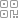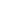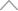6 2 9 1 1 1 8

# 相较神经网络，大名鼎鼎的傅里叶变换，为何没有一统函数逼近器？答案在这a0 + a1*sin(x) + b1*cos(x) + a2*sin(2x) + b2*cos(2x) + ...

a0 + a1*sin(x) + b1*cos(x) + c1*sin(y) + d1*cos(y) + a2*sin(2x) + b2*cos(2x) + c2*sin(2y) + d2*cos(2y) + e2*sin(x)*cos(y) + ...## 新知精选

IT研发▪2021年度十佳创作者

### 优选课程 新知学院

•扫码下载商业新知APP~

•回到顶部